1、多任务学习的网络结构的构造；

2、多任务学习对标的多目标优化的方法；2、others。。。。

MTL中的一个重大挑战源于优化过程本身。特别是，我们需要仔细平衡所有任务的联合训练过程， 以避免一个或多个任务在网络权值中具有主导影响的情况 。极端情况下，当某个任务的loss非常的大而其它任务的loss非常的小，此时多任务近似退化为单任务目标学习，网络的权重几乎完全按照大loss任务来进行更新，逐渐丧失了多任务学习的优势（具体优势可见：

https://zhuanlan.zhihu.com/p/361464660

https://zhuanlan.zhihu.com/p/361915151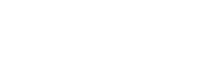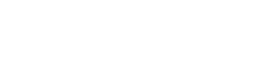1、loss大则梯度更新量也大；

2、不同任务的loss差异大导致模型更新不平衡的本质原因在于 梯度大小 ；

3、通过调整不同任务的loss权重wi可以改善这个问题；

4、直接对不同任务的梯度进行处理也可以改善这个问题；

1、在权重wi上做文章；

2、在梯度上做文章

### 1、Uncertainty Weighting

https://arxiv.org/pdf/1705.07115v3.pdf#### 在贝叶斯学习

https://book.douban.com/subject/26284941/

https://github.com/CamDavidsonPilon/Probabilistic-Programming-and-Bayesian-Methods-for-Hackers

https://github.com/CamDavidsonPilon/Probabilistic-Programming-and-Bayesian-Methods-for-Hackers

CamDavidsonPilon/Probabilistic-Programming-and-Bayesian-Methods-for-Hackers

https://github.com/CamDavidsonPilon/Probabilistic-Programming-and-Bayesian-Methods-for-Hackers

https://github.com/tensorflow/probability

https://github.com/pyro-ppl/pyro

https://zhuanlan.zhihu.com/p/56986840

1.偶然不确定性2.认知不确定性

（补充：异方差和同方差，以经典的线性回归为例，我们常常假设线性回归的误差项满足同方差，即误差项的方差是相同的，如果不相同则为异方差，一个比较形象的例子：

1 数据依赖性（异方差不确定性）依赖于输入数据，模型预测结果的残差的方差即随着数据的输入发生变化；

2、任务依赖性（同方差不确定性）是不依赖于输入数据的任意不确定性，它与模型输出无关，是一个在所有输入数据保持不变的情况下，在不同任务之间变化的量，因此，它可以被描述为与任务相关的不确定性，但是作者并没有详细解释在多任务深度学习中的同方差不确定性的严格定义， 而是认为同方差不确定性是由于任务相关的权重引起的 。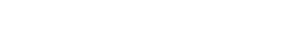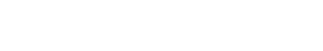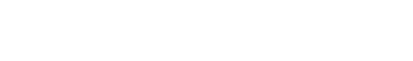（这个正比的公式是怎幺得到的。。。）

#### 现在让我们假设我们的模型输出由两个向量y1和y2组成，每个向量都遵循一个高斯分布：（这里作者没有说清楚，实际上这里作者是假设我们有两个回归型的目标任务，并且损失函数使用的是mse）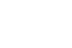````def criterion(y_pred, y_true, log_vars):`
`  loss = 0`
`  for i in range(len(y_pred)):`
`    precision = torch.exp(-log_vars[i])`
`    diff = (y_pred[i]-y_true[i])**2. ## mse loss function`
`    loss += torch.sum(precision * diff + log_vars[i], -1)`
`  return torch.mean(loss)````

``` ```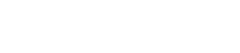git上对应的代码：

https://github.com/Mikoto10032/AutomaticWeightedLoss/blob/master/AutomaticWeightedLoss.py

````import torch`
`import torch.nn as nn`
```
```
`class AutomaticWeightedLoss(nn.Module):`
`    """automatically weighted multi-task loss`
`    Params：`
`        num: int，the number of loss`
`        x: multi-task loss`
`    Examples：`
`        loss1=1`
`        loss2=2`
`        awl = AutomaticWeightedLoss(2)`
`        loss_sum = awl(loss1, loss2)`
`    """`
`    def __init__(self, num=2):`
`        super(AutomaticWeightedLoss, self).__init__()`
`        params = torch.ones(num, requires_grad=True)`
`        self.params = torch.nn.Parameter(params) #parameters的封装使得变量可以容易访问到`
```
```
`    def forward(self, *x):`
`        loss_sum = 0`
`        for i, loss in enumerate(x):`
`            loss_sum += 0.5 * torch.exp(-log_vars[i]) * loss + self.params[i]`
`# +1避免了log 0的问题  log sigma部分对于整体loss的影响不大`
`        return loss_sum````

``` ```````from torch import optim`
`from AutomaticWeightedLoss import AutomaticWeightedLoss`
```
```
`model = Model()`
```
```
`awl = AutomaticWeightedLoss(2)  # we have 2 losses`
`loss_1 = ...`
`loss_2 = ...`
```
```
`# learnable parameters`
`optimizer = optim.Adam([`
`                {'params': model.parameters()},`
`                {'params': awl.parameters(), 'weight_decay': 0}`
`            ])`
```
```
`for i in range(epoch):`
`    for data, label1, label2 in data_loader:`
`        # forward`
`        pred1, pred2 = Model(data)  `
`        # calculate losses`
`        loss1 = loss_1(pred1, label1)`
`        loss2 = loss_2(pred2, label2)`
`        # weigh losses`
`        loss_sum = awl(loss1, loss2)`
`        # backward`
`        optimizer.zero_grad()`
`        loss_sum.backward()`
`        optimizer.step()````

``` ```

1、同方差不确定性衡量的定义方式是否合理；

2、如果在多任务学习中，我们主要是希望主任务的效果好，辅助任务的效果可能不是很care， 那幺如果恰好主任务是同方差不确定性最高的，则使用这种方法可能会削弱主任务的效果，这是最大的问题 ，因为这种处理的方式针对的是整个多任务模型的总体loss来设计的，无法满足对特定任务的不同程度的需求，因为作者原始的思路是不确定性越高的任务越应该削弱权重， 但是反过来想，不确定性越高的任务越难，如果我们反而让模型重点去学习这个任务，是否可以提高模型的能力 ；

3、 这里没有考虑权重和为1的问题，不过我觉得作者本来也没打算这幺做，影响不大，权重之和是否为1并不是问题其实，本来多任务也不一定需要权重为1的设定，另外权重简单做归一化就可以得到权重为1了。。

4、实际应用的一个问题，权重可能会变成负数，导致我们最终的loss变成负数了。。。也就是部分任务对于最终总loss的贡献是负贡献，我认为可能是这部分任务的不确定性太大使得模型训练困难，这个部分我们torch.relu进行截断就可以了

1、模型训练的效率低，最终运行时间由最复杂的任务决定；

2、复杂任务收敛的过程中，简单任务的局部最优权重可能会变差；